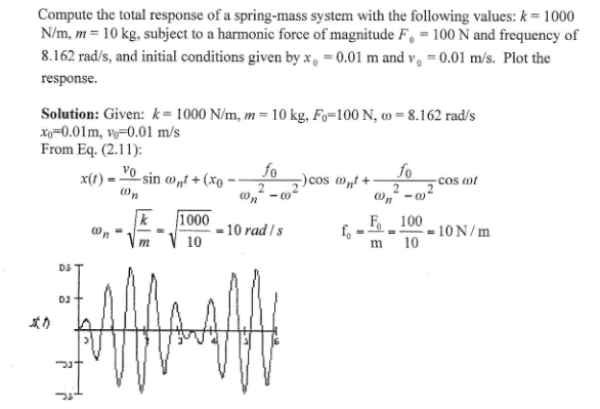Compute the total response of a spring-mass system with the following values: k=1000 N/m, m=10 kg, subject to a harmonic force of magnitude F0=100 N and frequency of 8.162 rad/s, and initial conditions given by x0=0.01 m and v0=0.01 m/s. Plot the response.Compute the total response of a spring-mass system with the following values: k=1000 N/m, m=10 kg, subject to a harmonic force of magnitude F0=100 N and frequency of 8.162 rad/s, and initial conditions given by x0=0.01 m and v0=0.01 m/s. Plot the response.

Vibrations Page 1 vibrations vibrations vibrations vibrations vibrations vibrations vibrations Vibrations Page 2